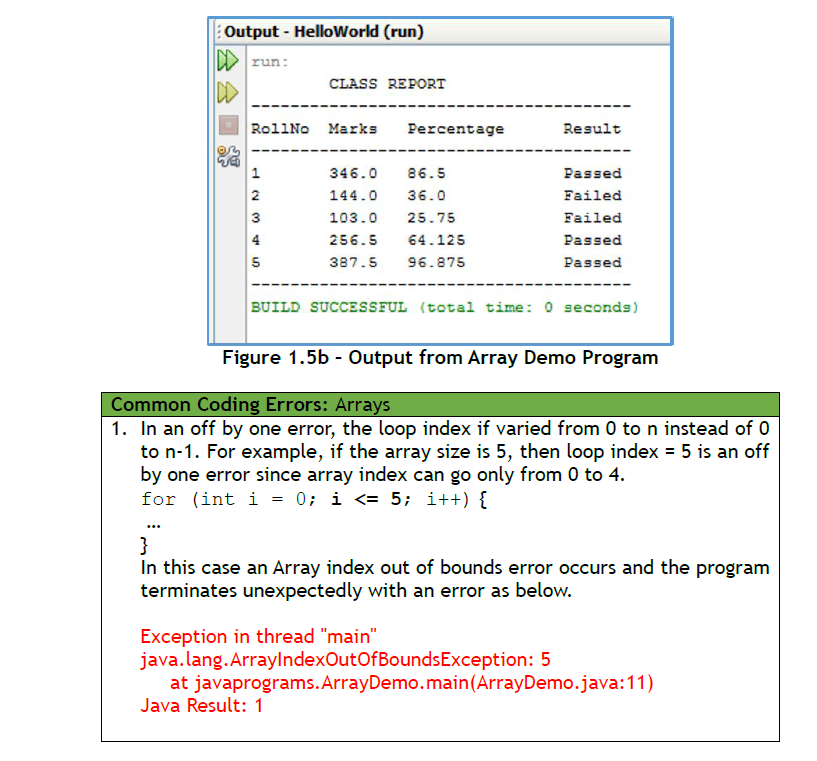Home » Online Computer Education » Learn Fundamentals of Java Programming “Arrays in Java Programming ” Lesson 15

# Learn Fundamentals of Java Programming “Arrays in Java Programming ” Lesson 15

Arrays

Previously you learned about variables that are place holders for a single value. Arrays are variables that can hold more than one value, they can hold a list of values of the same type. For example, to store the marks of a student, we used a variable:

double marks_obtained = 346;

To store the marks of another student, we changed the variable marks_obtained.

marks_obtained = 144;

By changing marks_obtained, we have lost information about the first student’s marks. What if we wanted to save the first student’s marks for later processing? We would need two variables, say marks1 and marks2. But, what if there were 5 students in the class. We would have to have 5 variables!

double marks1 = 346, mark2 144, marks3 243,
marks4=256.5, marks5 = 387.5;

Java provides a simpler way to store the marks of a 5 students. We use an array and define it as below:

double [] marks;

The [] brackets after the double data type tell the Java compiler that instead of a single value, the marks variable is an array that can hold more than one value, each of type double. To specify that the array can hold up to 5 marks, we specify the size of the array using the new keyword as shown below:

marks = new double [5 ];

The two statements – declaring an array and specifying its size can also be done in one statement.

double [] marks = new double ;

Now we can store five marks in the array, each element of the array is indexed by its position, starting from 0. So the five elements of the array are available at positions 0 to 4 as given below:

marks, 1], 2], 3], and marks

Note that the array index goes from 0 to n-1 for an array of size n and not from 1 to n. We can initialize the array statically (that is at compile time) as shown below:

double[] marks = {346, 144, 103, 256.5, 387.5} ;

The statement above creates an array of double of size 5, and elements are assigned with the numbers given in the curly braces. We can also assign the marks of students in the array dynamically (that is at runtime), we can write,

marks = 346;
marks = 144;
marks = 103;
marks = 256.5;
marks = 387.5;

To print all the marks, we can use a for loop, varying the loop index from 0 to 4.

for (int i = 0; < 5 ; i++)
System.out.println(marks[i]);

An array variable has a useful property, the length property, which is the size of the array.  For example, for the marks array, marks.length will be 5. We can thus code the previous for loop to print all the elements of an array as:

for (int i = 0; < marks.length; i++)
System.out.println(marks[i]);

The following code shows the use of an array to print the class report card for the five students.

for (int i = 0; < marks.length ; i++) {
double percentage = (marks[i]/total_marks)*100;
String result;
if (percentage >= 40)
result = “Passed”;
else
result = “Failed”;
System.out.print((i+1)+” \t” );
System.out.print( marks[i]+ “\t” );
System.out.print( percentage+” \t\t” );
System.out.println( result);
}

Notice the use of \t to print a tab between the numbers in the print statement. Also note that the roll number of the student has to be printed as (i+1) since the array index begins from 0 but roll numbers begin from 1. Figure 1.5a shows the complete program listing and Figure 1.5b displays the output from the Array Demo program.The main objective of this website is to provide quality study material to all students (from 1st to 12th class of any board) irrespective of their background as our motto is “Education for Everyone”. It is also a very good platform for teachers who want to share their valuable knowledge.